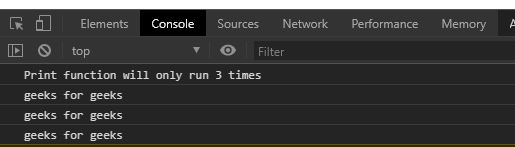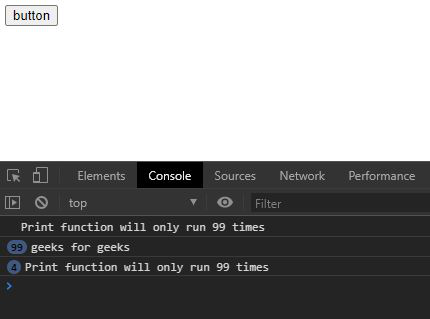Open in App
Not now

# Underscore.js _.before() Function

• Last Updated : 20 Dec, 2022

_.before() function in underscore.js is used to call a particular function a particular number of times. It uses a count variable that keeps the track of number of times the function is called.

Syntax:

`_.before(count, function)`

Parameters:

• Count: It is the number of times a function is to be called.
• Function: It is the function that runs the count times.

Return: This function returns  the count of the function call.

Note: Please Link the underscore CDN before using this code directly in the browser through the code.

Example 1:

## html

 ```<``html``>``  ``<``head``>``    ``<``script` `src = ``"https://cdnjs.cloudflare.com/ajax/libs/underscore.js/1.9.1/underscore-min.js" >``    ````  ````  ``<``body``>``    ``<``script``>``      ``let print=()=>{``        ``console.log("geeks for geeks")``      ``}``      ``console.log(`Print function will only run 3 times`)``      ``//the print function cannot run more than 3 times``      ``count=_.before(4, print);``      ``//calling count function more than 3 times``      ``count();``      ``count();``      ``count();``      ``count();``      ``count();``      ``count();``      ``count();``    ````  `````

Output:Example 2:

It is clear from the example that if The button is clicked again and again then print function will not be working as it as already reached the count.

## html

 ```<``html``>``  ``<``head``>``    ``<``script` `src = ``"https://cdnjs.cloudflare.com/ajax/libs/underscore.js/1.9.1/underscore-min.js" >``    ````   ````  ``<``body``>``    ``<``button` `id="btn">button``    ``<``script``>``      ``let print=()=>{``        ``console.log("geeks for geeks")``      ``}``      ``//the print function cannot run more than 99 times``      ``count=_.before(100, print);``      ``//calling count function more than 99 times``      ``let btn=document.querySelector("#btn");``      ``let run=()=>{``      ``console.log(`Print function will only run 99 times`)``        ``for(let i=0; i<``5000``; i++){``          ``count()``        ``}``      ``}``      ``btn.addEventListener("click", run)``    ````  `````

Output:My Personal Notes arrow_drop_up